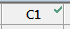# Formulas in columns

Formulas let you define data in a column as a function of data in other columns.

Formulas let you define data in a column as a function of data in other columns.

For example, if you record length in a column named L, and width in a column named W, you can use the formula W * L to automatically calculate the area and store it in a third column (named Area). When values in L or W change, the Area column is automatically updated.

You assign a formula to an entire column, not to individual cells. A formula assigned to a column cannot reference that same column directly or indirectly. For example, you cannot assign the formula 2 * C1 to column C1 because each data value in C1 cannot simultaneously be its original value and twice its original value.

###### Note

Columns with formulas have a green checkmark in the column number cell.## Assign a formula to a column

To assign a formula to a column follow these steps:
1. Click in the column.
2. Right-click in the worksheet and choose Formulas > Assign Formula to Column.
3. Enter the formula.
For more information about how to enter a formula, go to Create a formula with the Calculator.

### Example of assigning a formula to a column

Suppose you have data in C1 and C2. You want to calculate the sum of these two columns in C3, and the product of the two columns in C4. You want Minitab to automatically recalculate C3 and C4 whenever the data change in either C1 or C2.

1. Click in C3. Choose Formulas > Assign Formula to Column.
2. In Expression, type C1+C2. Click OK.
3. Click C4. Choose Formulas > Assign Formula to Column.
4. In Expression, type C1*C2. Click OK.
5. Verify that Formulas > Calculate All Formulas Automatically is selected.

## View the formulas assigned to columns

To view the formula for a column, hold the pointer over the formula icon in the column number cell. To view all the formulas in the worksheet, follow these steps.

1. Choose View > Command Line/History .
2. In the Command Line pane, type info, then press Run.

## Calculate formulas one time

You can control when formulas in a project are calculated.

###### Note

If formulas are not set to calculate automatically and the data in the formula change, the icon in the column number cell changes to a yellow triangle.

1. Right-click in the worksheet, then choose Formulas > Calculate All Formulas Automatically to deselect it.
2. To calculate all formulas in the project one time, choose Formulas > Calculate All Formulas Now. If this option is grayed out, all formulas are up to date.
###### Tip

To change the default settings for future sessions of Minitab, choose File > Options > Formulas.

## Delete a formula

1. Select the column or columns that contain the formulas to delete.
2. Choose Formulas > Remove Formulas from Selected Columns.
By using this site you agree to the use of cookies for analytics and personalized content.  Read our policy